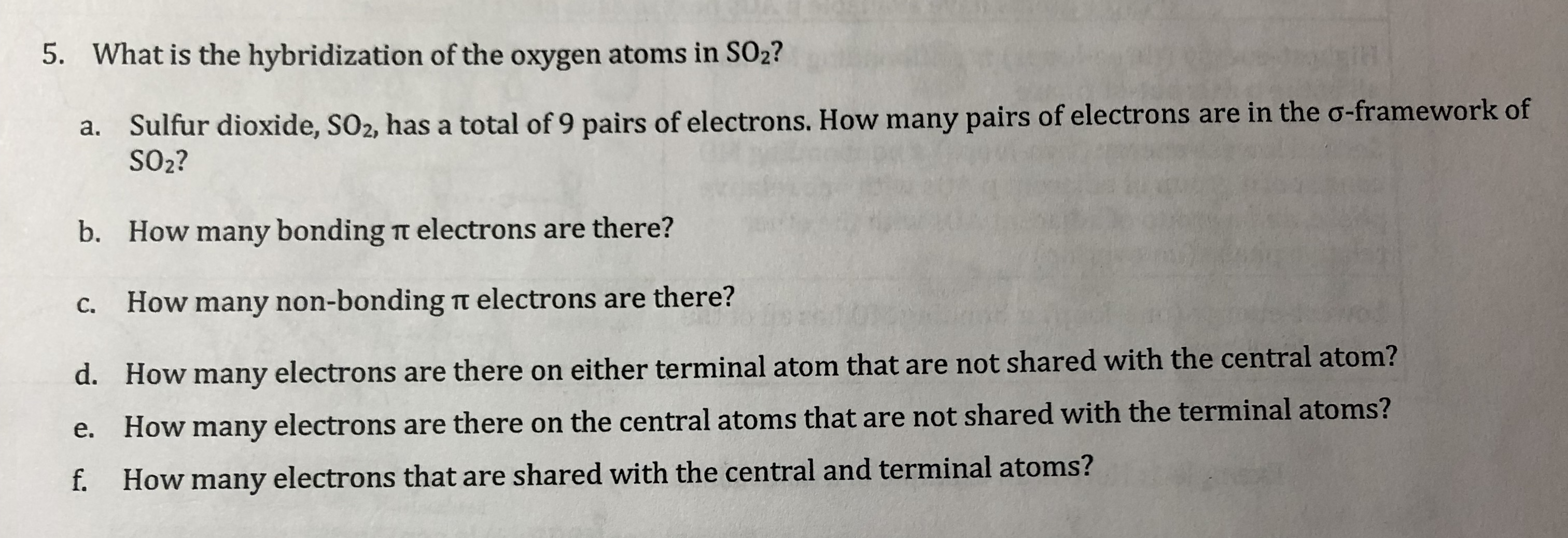# 5. What is the hybridization of the oxygen atoms in SO2? airs of electrons are in the o-framework of a. Sulfur dioxide, SO2, has a total of 9 pairs of electrons. How many p SO2? b. How many bonding π electrons are there? c. How many non-bonding π electrons are there? d. How many electrons are there on either terminal atom that are not shared with the central atom? e. How many electrons are there on the central atoms that are not shared with the terminal atoms? f. How many electrons that are shared with the central and terminal atoms?

Question

Can you please help me solve all parts of this problem? (5 a, b, c, d, e, and f)?help_outlineImage Transcriptionclose5. What is the hybridization of the oxygen atoms in SO2? airs of electrons are in the o-framework of a. Sulfur dioxide, SO2, has a total of 9 pairs of electrons. How many p SO2? b. How many bonding π electrons are there? c. How many non-bonding π electrons are there? d. How many electrons are there on either terminal atom that are not shared with the central atom? e. How many electrons are there on the central atoms that are not shared with the terminal atoms? f. How many electrons that are shared with the central and terminal atoms? fullscreen

2 Ratings

### Want to see the step-by-step answer?